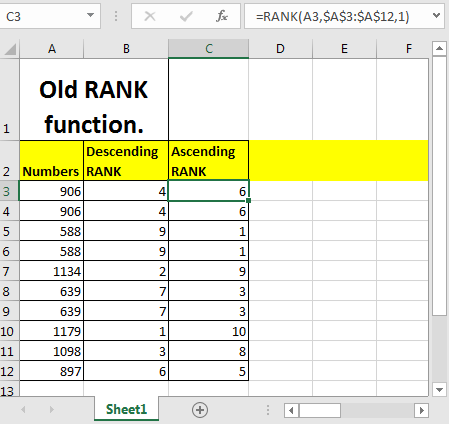# How to use the RANK function in Excel

The Rank Function in Excel is used to rank a number within a list. This function is outdated and excel does not recommend to use it. Excel has introduced two new ranking functions. The names are RANK.AVG and RANK.EQ. You can check them out here.Syntax of RANK Function

=RANK(number,ref_range,[order])

Number: The number that you want to rank
Ref_Range: The range in which you want to rank. The number must be in the ref_range.

[Order]: This argument is optional. 1 is for ascending ranking and 0 for descending. Ascending ranking means that higher number will get lover ranking. Descending ranking means a higher number will get a higher ranking. By default, it is Descending.
RANK FUNCTION EXAMPLE
So to rank each number in range A3:A12 in descending order we can write this RANK formula.

=RANK(A3,\$A\$3:\$A\$12)

Similarly, to rank each number in range A3:A12 in ascending order write this RANK formula.

=RANK(A3,\$A\$3:\$A\$12,1)

1 command excel to rank in ascending order.

The best thing about the RANK function that you don’t need to sort the data to rank.

This RANK function was used extensively in Excel 2007 and earlier. Now in Excel 2016 RANK.AVG and RANK.EQ is used.

Related Articles:

How to use the RANK.AVG Function in Excel

How to use the RANK.EQ Function in Excel

How to use the LARGE Function in Excel

How to use the RANK function in Excel

Sort numbers using Excel SMALL function in Excel

Popular Articles:

50 Excel Shortcuts to Increase Your Productivity

How to use the VLOOKUP Function in Excel

How to use the COUNTIF function in Excel

How to use the SUMIF Function in Excel

Terms and Conditions of use

The applications/code on this site are distributed as is and without warranties or liability. In no event shall the owner of the copyrights, or the authors of the applications/code be liable for any loss of profit, any problems or any damage resulting from the use or evaluation of the applications/code.